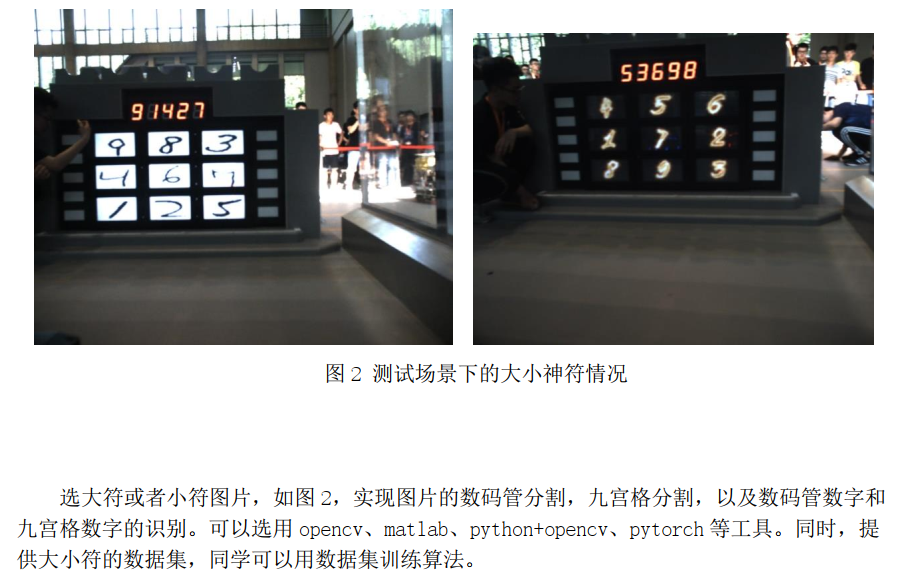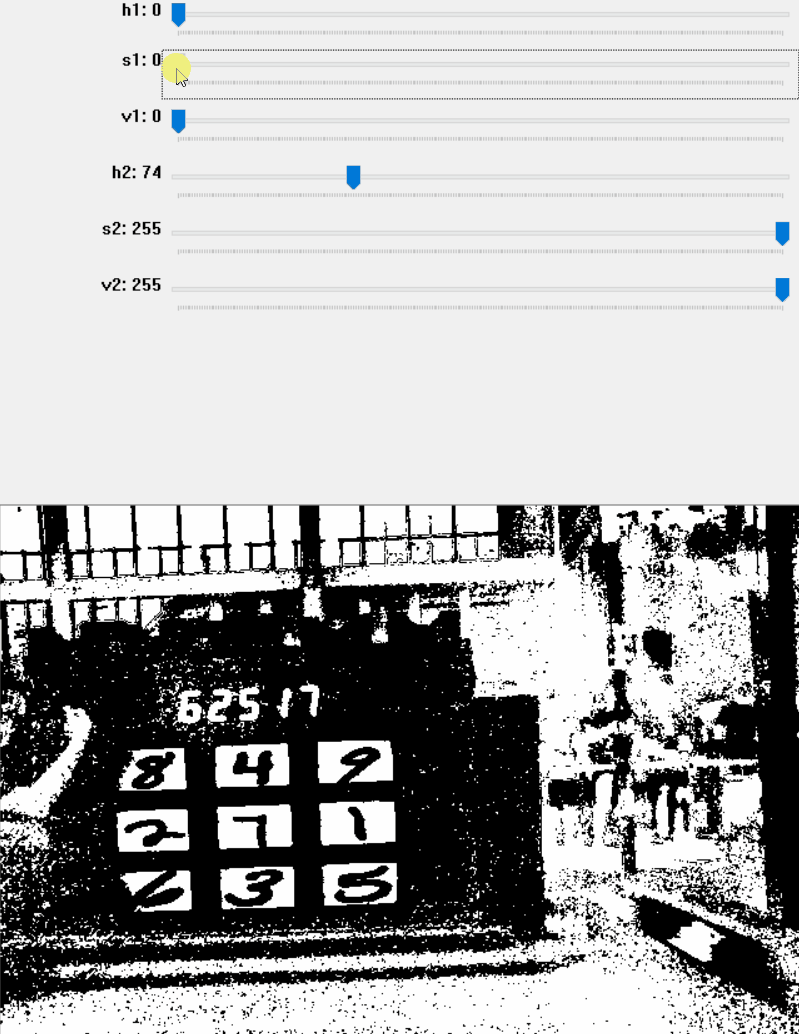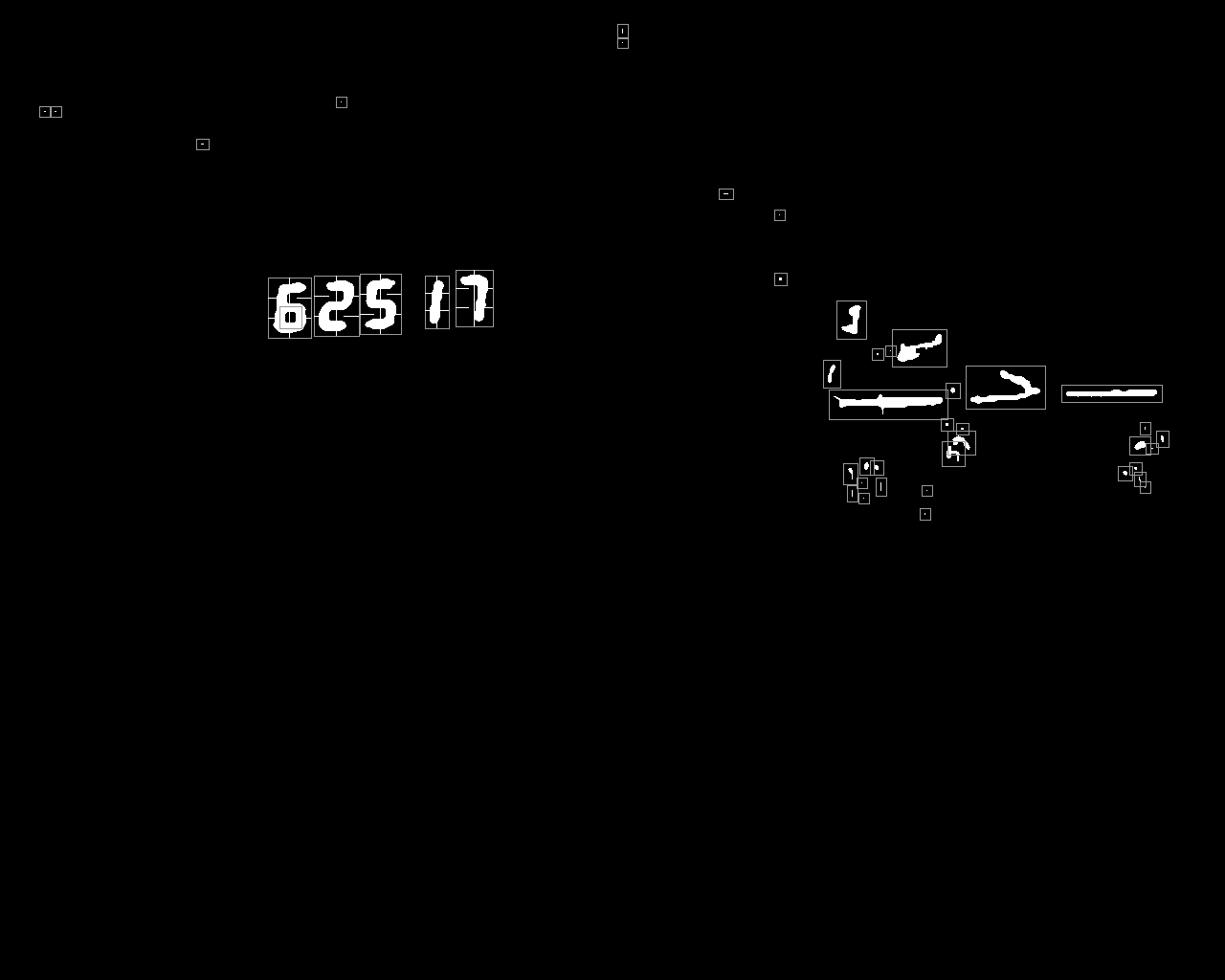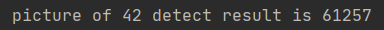# 一、问题# 三、代码

1.转换成hsv格式，利用hsv格式进行筛选，其中筛选参数lower，upper需要根据自己情况来设定。

``````hsv = cv2.cvtColor(image_org, cv2.COLOR_BGR2HSV)

lower = np.array([h0, s0, v0])
upper = np.array([h1, s1, v1])

image_gray = cv2.inRange(hsv, lower, upper)``````2.对hsv图像进行高斯滤波，二值化处理

``````image_gray = cv2.GaussianBlur(image_gray, (7, 7), 0)
ret, image_bin = cv2.threshold(image_gray, 200, 255, cv2.THRESH_BINARY)``````

3.进行形态学处理，利用闭运算处理。

``cv2.morphologyEx(image_bin, cv2.MORPH_CLOSE, kernel2, iterations=1)``

4. 运用cv2.findContours得到边界，下图是可视化边界的样图。5.对上述的得到的边框进行位置以及大小的筛选，进行穿线法识别，下面是穿线法的代码。

``````def TubeIdentification(filename, num, image):
tube = 0
tubo_roi = [
[image.shape * 0/3, image.shape * 1/3, image.shape * 1/2,
image.shape * 1/2],
[image.shape * 1/3, image.shape * 1/3, image.shape * 2/3,
image.shape - 1  ],
[image.shape * 2/3, image.shape * 2/3, image.shape * 2/3,
image.shape - 1  ],
[image.shape * 2/3, image.shape -1   , image.shape * 1/2,
image.shape * 1/2],
[image.shape * 2/3, image.shape * 2/3, image.shape * 0/3,
image.shape * 1/3],
[image.shape * 1/3, image.shape * 1/3, image.shape * 0/3,
image.shape * 1/3],
[image.shape * 1/3, image.shape * 2/3, image.shape * 1/2,
image.shape * 1/2]]
i = 0
while(i < 7):
if(Iswhite(image, int(tubo_roi[i]), int(tubo_roi[i]),
int(tubo_roi[i]),int(tubo_roi[i]))):
tube = tube + pow(2,i)

cv2.line(image, ( int(tubo_roi[i]),int(tubo_roi[i])),
(int(tubo_roi[i]), int(tubo_roi[i])),
(255,0,0), 1)
i += 1

if(tube==63):
onenumber = 0
elif(tube==6):
onenumber = 1
elif(tube==91):
onenumber = 2
elif(tube==79):
onenumber = 3
elif(tube==102 or tube==110):
#110是因为有干扰情况
onenumber = 4
elif(tube==109):
onenumber = 5
elif(tube==125):
onenumber = 6
elif(tube==7):
onenumber = 7
elif(tube==127):
onenumber = 8
elif(tube==103):
onenumber = 9
else:
onenumber = -1

cv2.imwrite(filename + '_' + str(num) + '_' + str(onenumber) + '.png', image)
return onenumber

def Iswhite(image, row_start, row_end, col_start, col_end):
white_num = 0
j=row_start
i=col_start

while(j <= row_end):
while(i <= col_end):
if(image[j][i] == 255):
white_num+=1
i+=1
j+=1
i=col_start
#print('white num is',white_num)
if(white_num >= 5):
return True
else:
return False
``````

6.最终得到结果（未按顺序输出）# 五、参考

https://blog.csdn.net/zong596568821xp/article/details/85098590

https://blog.csdn.net/km2km2km/article/details/94778305

https://blog.csdn.net/sandalphon4869/article/details/95398033

https://blog.csdn.net/spw_1201/article/details/78310349

### SET ROWCOUNT 1 与 TOP(1) 的区别_光义的博客-程序员秘密

SET ROWCOUNT  1 与 TOP(1) 的区别 都能实现只取得一条语句。。 使 SQL Server 在返回指定的行数之后停止处理查询。 Transact-SQL 语法约定语法 SET ROWCOUNT { number | @number_var } 参数number | @numbe

### BZOJ1861 [Zjoi2006]Book 书架_weixin_30505225的博客-程序员秘密

Splay维护操作。学习了一个很好的remove操作。 1 #include&lt;bits/stdc++.h&gt; 2 using namespace std; 3 const int N=8e4+5; 4 const int inf=1e9; 5 int fa[N],c[N],size[N],pos[N],a[N],v[N],n,m,rt; ...